﻿ Simulation of a Silage Container Wind Loading

### Simulation of a Silage Container Wind Loading

Ladislav NovotnýOPEN ACCESSPEER-REVIEWED

## Simulation of a Silage Container Wind Loading

Ladislav NovotnýDepartment of Applied Mechanics and Mechatronics, Technical University of Košice, Košice, Slovakia

### Abstract

Loading a construction with the wind is one of many load conditions for the steel constructions. For its simulation, many approaches are available. In the article, a method is described that is based on the loading set by the EU standard, as well as on the simulation of air flow around the container by the finite element method. In the approach set by the norm, the loading changes according to the function which is dependent on the point defined by the angle of the polar coordinate system. In the approach based on the flow simulation by the finite element method, the air pressure is calculated, which affects every finite element of the container. The contribution includes both approaches used in the silage container wind loading; their application is described in detail.

123
Prev Next

### Cite this article:

• Novotný, Ladislav. "Simulation of a Silage Container Wind Loading." American Journal of Mechanical Engineering 1.7 (2013): 204-207.
• Novotný, L. (2013). Simulation of a Silage Container Wind Loading. American Journal of Mechanical Engineering, 1(7), 204-207.
• Novotný, Ladislav. "Simulation of a Silage Container Wind Loading." American Journal of Mechanical Engineering 1, no. 7 (2013): 204-207.

 Import into BibTeX Import into EndNote Import into RefMan Import into RefWorks

### 1. Introduction

A silage container is used for conserving and storing the grass crops by the method of silage. Silage is a way of keeping the crops in juicy form with the help of lactic fermentation anaerobic bacteria. The whole procedure is done without access of air so that the nutriments and vitamins are preserved. The silage is then a final product. The most common crops are corn, wheat, barley, pea, etc.

Figure 1 shows the silage container 16.1 m high and with the diameter of 3.7 m. The silage container must comply with the numerous requirements and standards which stipulate the container loadings such as the self-weight loading, the silage loading, the wind loading, the snow loading, the loading of the walls during filling up and storage, the loading of the walls during clearing of, and a limiting state of the silage container buckling. The contribution also describes an option of simulation of the wind loading when applying the finite element method.

### 2. The Silage Container Wind Loading

When simulating the silage container wind loading, it is possible to use the loading set by the standards. A different approach must be applied when simulating air flow around the container and the consequent calculation of the pressures which affect the container in a stress analysis of the container.

2.1. Application of the Wind Loading Defined by the EU Standard

European standard ENV 1993-4-1, appendix D, describes the wind loading along the circuit of a freestanding container as a function of angular coordination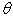with a start at windward side (Figure 2).

Coefficient of distribution of the wind pressure  along the freestanding closed roof container is represented by the relation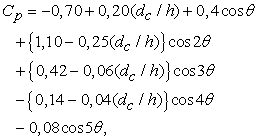(1)

where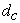is a diameter of the container,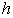is an overall weight,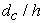is a ratio between the main measures. If the container is not closed with the roof, it is possible to add to the values of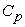, according to the relation (1), the following constant values of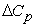, which diminish the figures of the wind pressure at the windward side

a) for open containers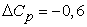,

b) for vented containers with small openings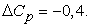According to the standard STN 1991-1-4, the wind pressure is then defined by the relation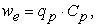(2)

where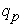is the high wind pressure andis distribution of the wind pressure along the circuit of the freestanding container. The high wind pressure is calculated from the relation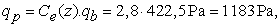where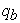is the basic wind pressure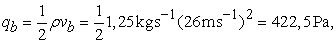(3)

where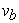is the basic wind velocity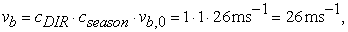where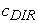is the directivity coefficient (page 18/3)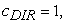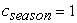is the season coefficient (page 18/1) ,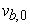is a fundamental value of the basic wind velocity defined by the geographical map (page 16, Tab. NB3)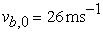,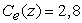is the wind exposure coefficient for surfaces (for the terrain of the II. category) (page 22, Figure 4) .

Figure 3 shows the process of the function of the wind pressure distributionalong the circuit of the container with the measures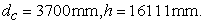This functionis shown in the Figure 3 in dependence on the polar coordinate of the angle, defined in the Figure 2. A great number of the finite element systems allow putting of the function of the wind distribution only in the Cartesian coordinate system. In using the transformation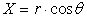(4)

we can display thefunction in dependence on the Cartesian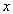coordinate, as shown in the Figure 4, while it is held that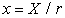.

Figure 4. The function of distribution inin dependence on thecoordinate

In order to prevent creating of a complicated macro, a great number of the finite element systems have the option of putting the pressure function in the form of polynomial . In the applied system, there was the option of using the second degree polynomial. For this reason, thefunction was approximated through the second degree polynomial .

For achieving the best possible correlation, the function was divided into four parts, and the individual parts were approximated through the second degree polynomial. Divisions of the domain of- coordinates were performed so that it would respond to the division of the anglein the polar coordinate system into the intervals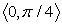,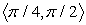,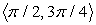,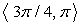.

Figure 5. Division of thefunctions into the subregion and their approximation

The function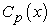for the domain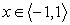, which responds to the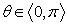in the individual subintervals, is approximated in the forms

for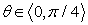in the form of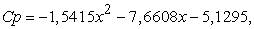for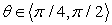in the form of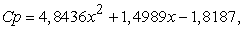for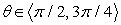in the form of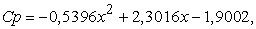for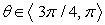in the form of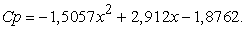The function of the wind distribution approximated in the above mentioned way could be thus put as the wind pressure on the silage container walls according to the Eqv. 2 and with the use of the transformation Eqv. 4.

2.2. Simulation of Air Blast around the Silage Container

In simulating air blast around the silage container, one of the boundary conditions was formed by the wind velocity calculated from the Eqv.3. Another condition was represented by the wind pressure in the point of the imagined pipe output. The Figure 4, Figure 5 and Figure 6 show the results of the flow simulation - the process of the air blast velocity around the container.

The colour velocity scale in the Figure 5 and the Figure 6 is equal to the one in the Figure 4. The pressures calculated in the flow simulation were used as the load conditions in the mechanical analysis of stress stage in the silage container.

### 3. The Mechanical Analysis from the Wind Loading

The load boundary condition in the wind loading is represented by the pressure affecting every finite element calculated in the flow simulation. The prescribed geometrical conditions are presented by the prescribed zero displacement in the points of fixation of the stand of the silage container.

The results of the static analysis from the wind loading are shown in the Figure 9 on the construction displayed in the deformed shape in the scale.

From the Figure 9 it is obvious that it would be suitable to strengthen the stands of the silage container and with the help of the ring along the whole circuit in the point of the stand fixation to eliminate the concentration of the stress around the point of the joint.

### 4. Conclusion

The article presents calculation of the stress in the silage container from the wind loading. However, as it was mentioned, the silage container is loaded by other loadings as well, such as the self-weight loading, the silage loading, the snow loading, the loading of the walls during filling up and storage, the loading of the walls during clearing of, or a limiting state of the silage container buckling . Since many of them may work simultaneously with the wind loading, the complex analysis is necessary for evaluating the container strength . In linear static analysis, the linear combination of individual types of loading can be performed. However, the silage container is a thin shell construction and thus it is necessary to evaluate its buckling behaviour - by the nonlinear analysis . In such a case, it is not possible to use the principle of superposition.

### Acknowledgement

This research was supported by the of the Ministry of Education of under grant 1/0090/12.

### References

  Cabúk,P., Vehec,, Slosarčík,S., Meassuring of pressure in intra-abdomen, In: Recent Advances in Numerical Modelling. : Electrotechnical Institute, 2009, pp. 162-166.In article  Fedorko, G., Determination of material characteristics of pipe conveyor belts for mathematical modeling. Doprava a logistika (CD-ROM), Vol. 9, 2011, pp. 474-483.In article  Ionescu,Th., Standardization of Terminology, Mechanism and Machine Theory. Vol. 38, nos. 7-10, 2003, pp. 598-1111.In article  Ostertagová, O., Modelling using polynomial regression, Procedia Engineering, Vol. 48, 2012, p. 500-506.In article CrossRef  Sadovský,Z., Kriváček,J., Ivančo, V.,Ďuricová, A., Computational modelling of geometric imperfections and buckling strength of cold-formed steel, Journal of Constructional Steel Research. Vol. 78, 2012, pp. 1-7.In article CrossRef  Semrád, K., Fatigue and Buckling Strength Analysis of the Wing Hinge in the Creo Simulation Environment, Journal of Mechanics Engineering and Automation. Vol. 3, no. 4, p. 247-250.In article  STN EN 1991 – 1 – 4 Common loading, wind load.In article